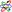## Convective Derivative

A Derivative taken with respect to a moving coordinate system, also called a Lagrangian Derivative. It is given bywhereis the Gradient operator andis the Velocity of the fluid. This type of derivative is especially useful in the study of fluid mechanics.When applied to,